Type Here to Get Search Results !

# MATHEMATICS FORM ONE TOPIC 4: UNITS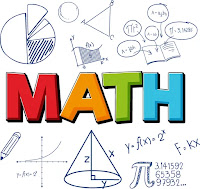UNITS
A unit – is defined as a symbol or sign which is assigned to a number to describe a kind of measurement made
Convertion of One Unit of Length to Another
Convert one unit of length to another
The conversion of one unit to another is done by considering the arrangement below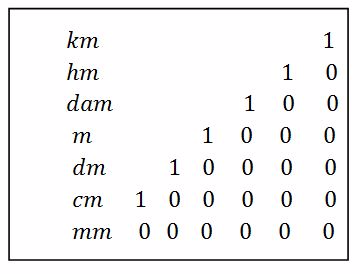Example, from the above we get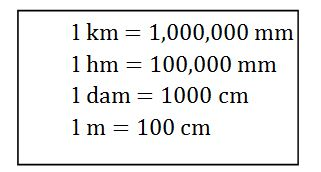Computations on Metric Units of Length
Perform computations on metric units of length
Example 1
Convert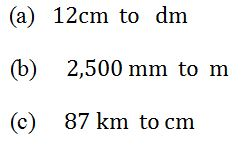Solution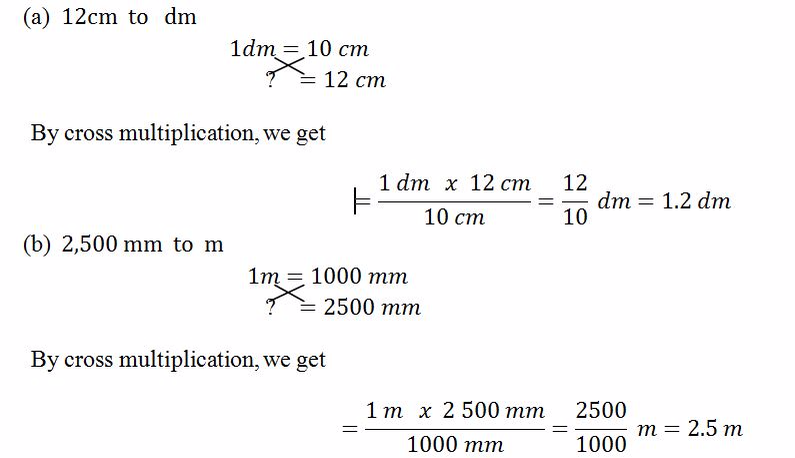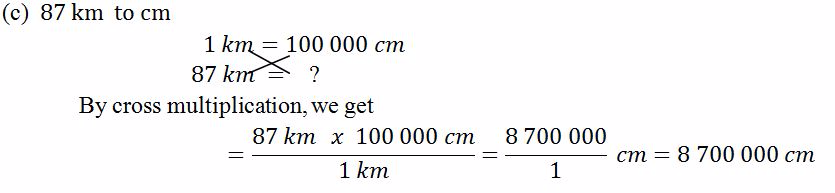Convertion of One Unit of Mass to Another
Convert one unit of mass to another
The conversion of one unit to another is done by considering the arrangement below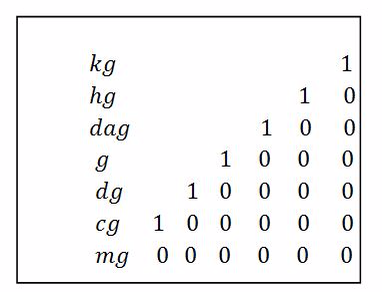Example, from the above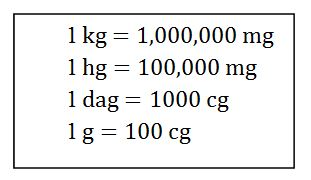The conversion of tonneto other units is done converting it to kilogramfirst and then fromkilogramto the required unit.
1 tonne = 1000kg
Computation on Metric Units of Mass
Perform computation on metric units of mass
Example 2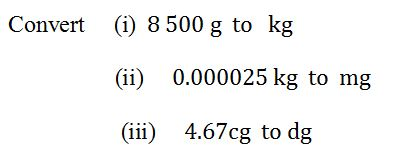Solution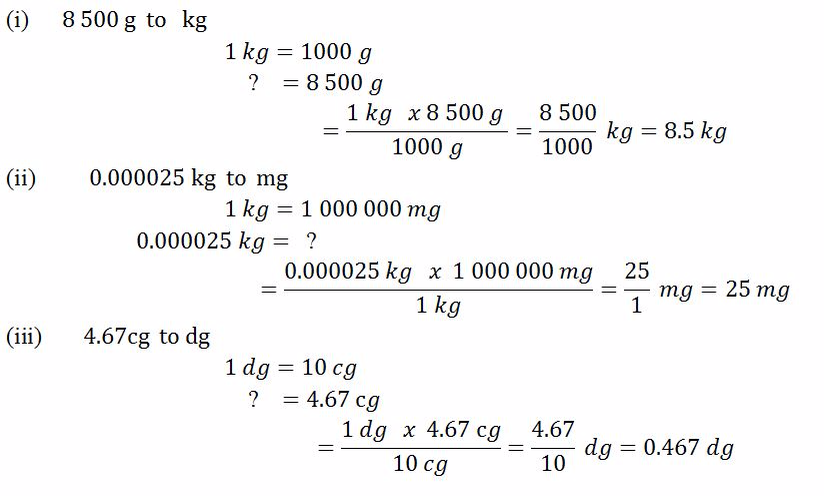The units of time are of two types, smaller and larger units of time.Smaller units of time includes seconds, minutes, hours and days.Larger units of time includes week, month, year, decade, century,millennium.
Convertion of One Unit of Time to another
Convert one unit of time to another
The conversion of units of time to another can be done by considering the arrangement below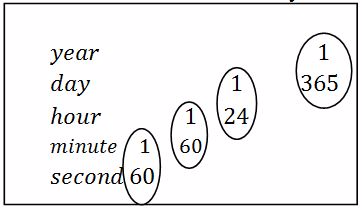Example, from the circle above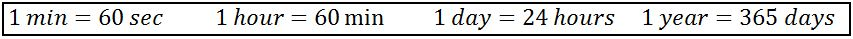Also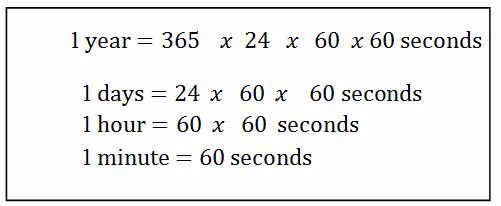Example 3
Convert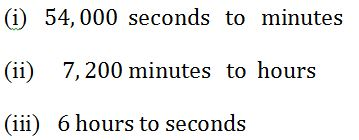Solution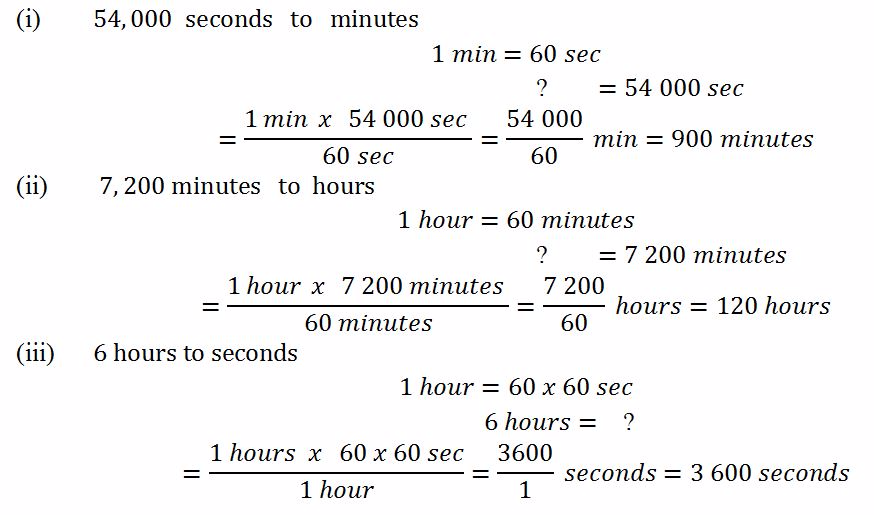Convertion of Unit Time of 12 Hour Clock to 24 Hour Clock and Vice Versa
Read and convert unit time of 12 hour clock to 24 hour clock and vice versa
The hours can exist in two systems: 12- hour clock and 24 - hour clock.
A 12- hour clock has 12 hours between midnight and midday(a.m)and 12 hours between midday and midnight(p.m).
A 21 - hour has 24 hours in a day.Times in the morning are the same in both systems.For times in the afternoon, convert by adding or subtracting 12 hours
Example 4
Convert the following times from the 12 - hour clock to 24 - hour clock.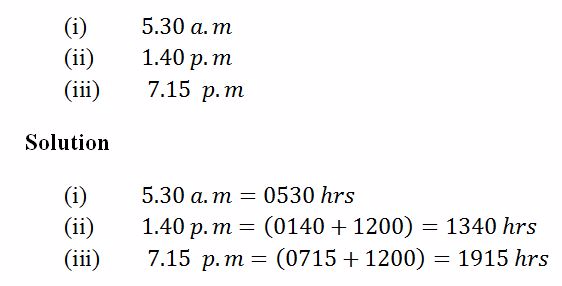Example 5
Convert the following times from the 24 - hour clock to 12 - hour clock.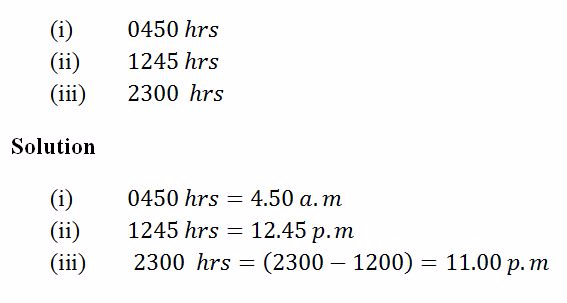Standard Unit of Measuring Capacity
State the standard unit of measuring capacity
Capacity is related to the volume.
Definitions:
• Capacity-is defined as the ability hold or contain something
• The S.I unit of capacity is litre.
• Volume –is defined as the amount of space occupied by a substance
• The S.I unit of volume is cubic metres (m3)
Capacity is related to the volume as follows:
1 litre = 1000cm3 = 0.001m= 1dm3
Also 1 ml = 1 cm3
Other units related to litre are kiloliter (kl), hectoliter (hl), decalitre (dal), litre (l), deciliter (dl), centiliter (cl) and mililitre (ml).
The conversion of one unit to another is done by considering the arrangement below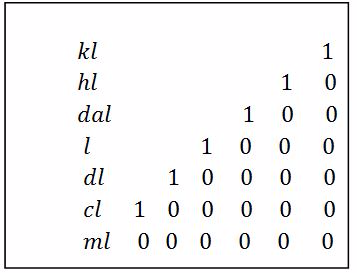Example, from the above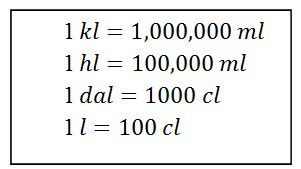The Litre in Daily Life
Use the litre in daily life
Example 6
Convert the following units into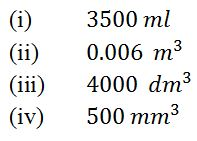Solution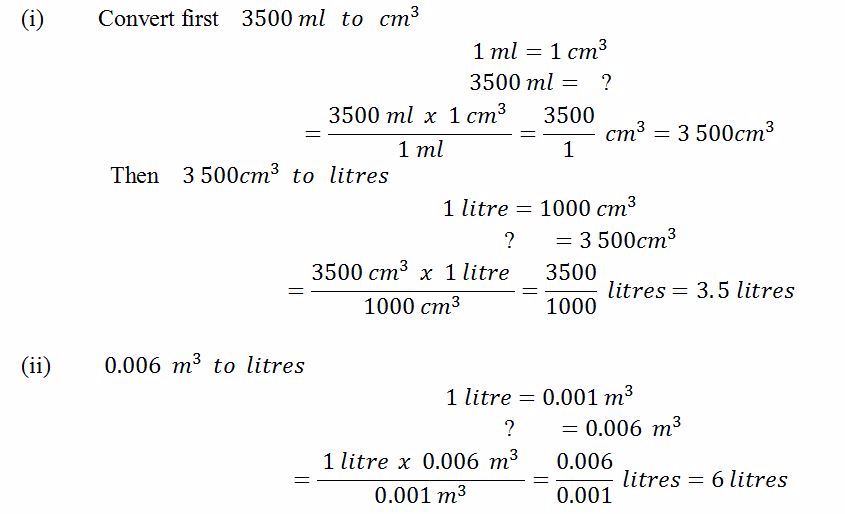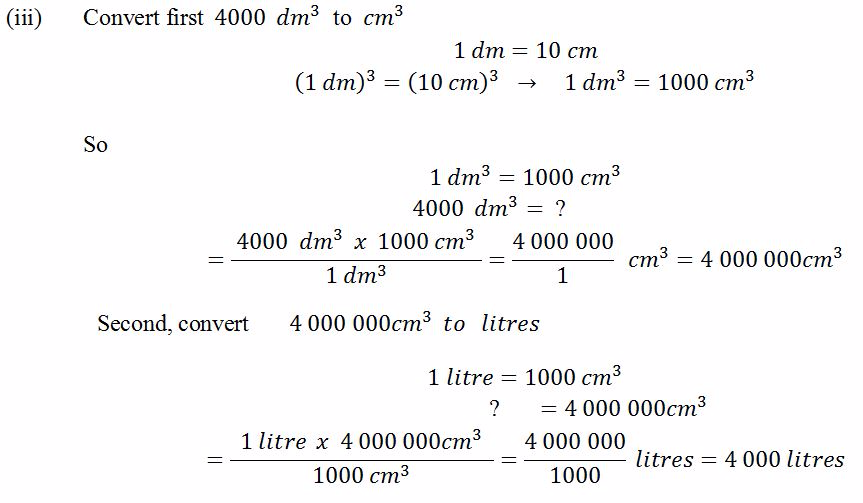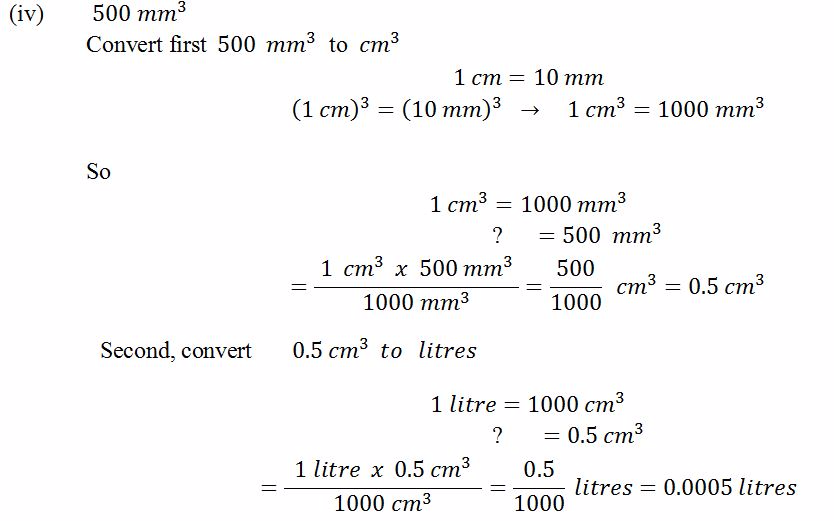Tags